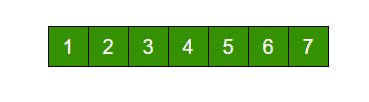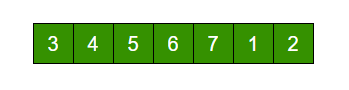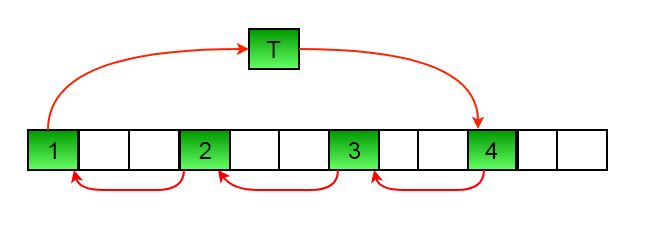Related Articles

# Java Program For Array Rotation

• Last Updated : 03 Mar, 2021

Write a function rotate(ar[], d, n) that rotates arr[] of size n by d elements.Rotation of the above array by 2 will make arrayMETHOD 1 (Using temp array)

```Input arr[] = [1, 2, 3, 4, 5, 6, 7], d = 2, n =7
1) Store d elements in a temp array
temp[] = [1, 2]
2) Shift rest of the arr[]
arr[] = [3, 4, 5, 6, 7, 6, 7]
3) Store back the d elements
arr[] = [3, 4, 5, 6, 7, 1, 2]```

Time complexity : O(n)
Auxiliary Space : O(d)

METHOD 2 (Rotate one by one)

```leftRotate(arr[], d, n)
start
For i = 0 to i < d
Left rotate all elements of arr[] by one
end```

To rotate by one, store arr in a temporary variable temp, move arr to arr, arr to arr …and finally temp to arr[n-1]

Let us take the same example arr[] = [1, 2, 3, 4, 5, 6, 7], d = 2
Rotate arr[] by one 2 times
We get [2, 3, 4, 5, 6, 7, 1] after first rotation and [ 3, 4, 5, 6, 7, 1, 2] after second rotation.

## Java

 `class` `RotateArray ``{``    ``/*Function to left rotate arr[] of size n by d*/``    ``void` `leftRotate(``int` `arr[], ``int` `d, ``int` `n) ``    ``{``        ``int` `i;``        ``for` `(i = ``0``; i < d; i++)``            ``leftRotatebyOne(arr, n);``    ``}`` ` `    ``void` `leftRotatebyOne(``int` `arr[], ``int` `n) ``    ``{``        ``int` `i, temp;``        ``temp = arr[``0``];``        ``for` `(i = ``0``; i < n - ``1``; i++)``            ``arr[i] = arr[i + ``1``];``        ``arr[i] = temp;``    ``}`` ` `    ``/* utility function to print an array */``    ``void` `printArray(``int` `arr[], ``int` `size) ``    ``{``        ``int` `i;``        ``for` `(i = ``0``; i < size; i++)``            ``System.out.print(arr[i] + ``" "``);``    ``}`` ` `    ``// Driver program to test above functions``    ``public` `static` `void` `main(String[] args) ``    ``{``        ``RotateArray rotate = ``new` `RotateArray();``        ``int` `arr[] = {``1``, ``2``, ``3``, ``4``, ``5``, ``6``, ``7``};``        ``rotate.leftRotate(arr, ``2``, ``7``);``        ``rotate.printArray(arr, ``7``);``    ``}``}`` ` `// This code has been contributed by Mayank Jaiswal`

Output :

```3 4 5 6 7 1 2
```

Time complexity : O(n * d)
Auxiliary Space : O(1)
METHOD 3 (A Juggling Algorithm)
This is an extension of method 2. Instead of moving one by one, divide the array in different sets
where number of sets is equal to GCD of n and d and move the elements within sets.
If GCD is 1 as is for the above example array (n = 7 and d =2), then elements will be moved within one set only, we just start with temp = arr and keep moving arr[I+d] to arr[I] and finally store temp at the right place.

Here is an example for n =12 and d = 3. GCD is 3 and

```Let arr[] be {1, 2, 3, 4, 5, 6, 7, 8, 9, 10, 11, 12}

a)    Elements are first moved in first set – (See below diagram for this movement)arr[] after this step --> {4 2 3 7 5 6 10 8 9 1 11 12}

b)    Then in second set.
arr[] after this step --> {4 5 3 7 8 6 10 11 9 1 2 12}

c)    Finally in third set.
arr[] after this step --> {4 5 6 7 8 9 10 11 12 1 2 3}
```

## Java

 `class` `RotateArray ``{``    ``/*Function to left rotate arr[] of size n by d*/``    ``void` `leftRotate(``int` `arr[], ``int` `d, ``int` `n) ``    ``{``        ``int` `i, j, k, temp;``        ``for` `(i = ``0``; i < gcd(d, n); i++) ``        ``{``            ``/* move i-th values of blocks */``            ``temp = arr[i];``            ``j = i;``            ``while` `(``true``) ``            ``{``                ``k = j + d;``                ``if` `(k >= n) ``                    ``k = k - n;``                ``if` `(k == i) ``                    ``break``;``                ``arr[j] = arr[k];``                ``j = k;``            ``}``            ``arr[j] = temp;``        ``}``    ``}`` ` `    ``/*UTILITY FUNCTIONS*/``     ` `    ``/* function to print an array */``    ``void` `printArray(``int` `arr[], ``int` `size) ``    ``{``        ``int` `i;``        ``for` `(i = ``0``; i < size; i++)``            ``System.out.print(arr[i] + ``" "``);``    ``}`` ` `    ``/*Function to get gcd of a and b*/``    ``int` `gcd(``int` `a, ``int` `b) ``    ``{``        ``if` `(b == ``0``)``            ``return` `a;``        ``else``            ``return` `gcd(b, a % b);``    ``}`` ` `    ``// Driver program to test above functions``    ``public` `static` `void` `main(String[] args) {``        ``RotateArray rotate = ``new` `RotateArray();``        ``int` `arr[] = {``1``, ``2``, ``3``, ``4``, ``5``, ``6``, ``7``};``        ``rotate.leftRotate(arr, ``2``, ``7``);``        ``rotate.printArray(arr, ``7``);``    ``}``}`` ` `// This code has been contributed by Mayank Jaiswal`

Output :

```3 4 5 6 7 1 2
```

Time complexity : O(n)
Auxiliary Space : O(1)

Please refer complete article on Program for array rotation for more details!

My Personal Notes arrow_drop_up